|

# 坐北朝南的房屋为啥好?其他朝向有啥讲究?

辛辛苦苦打拼多年，终于将新房买到了手，除了希望能够让自己住的更加的舒适之外，同时也希望这套房屋能够拥有一个良好的风水，从而让自己的运势兴旺起来。而站在风水的角度来看。房屋的朝向，与风水的联系是相当紧密的。接下来就跟着小编一起来了解一下。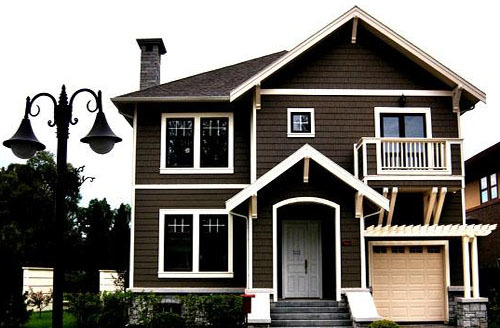房屋朝向风水

房屋朝向风水：坐北朝南

坐北朝南的房屋从古至今都是公认的最好的朝向的房屋，古代的正房都是朝南方向，这是为什么呢？这是和在北半球，阳光的照射角度息息相关的。首先，朝南的房屋在冬天阳光也一样能照射到同样的深处，令人有明亮温暖的感觉，而夏季阳光直射，屋内则受不到强烈日光的照射。

房屋朝向风水：其他可选朝向

正南方向当之无愧是首选，但条件所限时，我们还是可以选择以下朝向（按优劣顺序顺次排列）：东南、东、西南、北和西。虽然北向和西向的房屋接受日照的时间比较少，但是只要有2-3小时接受日照就会消除房间内湿气，杀灭细菌，调节室内温度。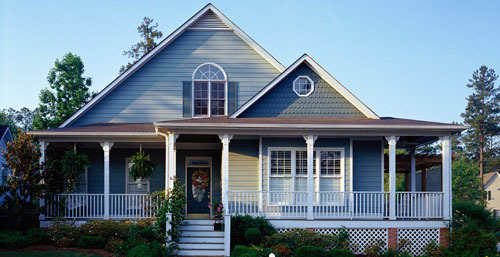其他朝向的房屋风水解析

坐东朝西

坐东朝西的房子使得主人身体康健、家庭幸福，但美中不足的是事业方面不是很让人满意，事业比较衰败，有志难申。坐西朝东的房子会使得家里显得生气勃勃，同时也是一个适合创业的流年方位。但家居这个方位，在流年上不是很吉利，中年男子健康多忧。所以这个方位房子的主人要多注意了。

坐东南朝西北

坐东南朝西北的房子的主人要特别注意了，这个房子的朝向是不吉之象，尤其不利于中年女人，会使得家庭不和，且财运受阻。如果你还没有买房的话就趁早换一家，如果买了就想想有什么方法可以化解吧。坐西北朝东南的房子是努力进取之象，有小成但也有小忧。平平淡淡的过着小日子之家。

坐西南朝东北

坐西南朝东北的房子这是一个相对吉祥的人居风水方位。内在和美，外在平实，是非常适合家庭居住的流年方位。坐东北朝西南的房子也属于大吉之象，是非常适合办公环境的流年方位，宜创业，多有发财升迁之喜。所以，这个方位适合创业办公选择，如果你正在为自己公司选择办公地，一定要记得方位要坐东北朝西南哦。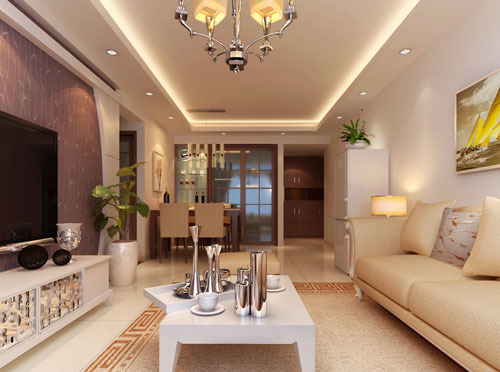家居功能区朝向解析

客厅卧室朝向

房屋内多个房间不可能同时朝着一个方向，这时，应当以客厅和卧室的朝向为主要考虑对象，即朝南方向。可是大多数家庭拥有不仅一个卧室，该怎么选呢？应以主卧室朝向或多数卧室朝向为评判标准，卧室以南向为佳，但最好不要靠外墙，朝向为东南、东、东北也可，但最好不要选朝西卧室。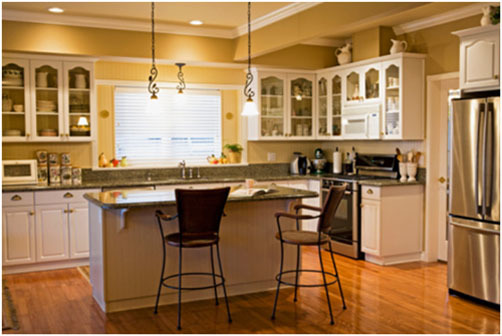厨卫朝向

卫生间比较潮湿，易滋生病菌，要保证采光、通风，所以要避免西向的卫生间。厨房会产生许多油烟，而且形成时间短，一般会用抽油烟机或是排气扇向外排气。由于北方冬季西北风比较多，风压太大会引起油烟“倒灌”，所以厨房最好不要选择北向。房屋其他风水禁忌

1、尖锐立物，冲煞严重

尖锐多角的大型景观雕塑即使是立于公共场所，如果是在自家住宅附近，冲煞亦很严重，尤其是近距离内冲到门窗者更甚。

2、住宅忌建于天桥旁边

高架路或天桥边，位于这些地方的房屋，经常饱受噪音及长期的震动，易造成精神衰落；如果又位于天桥或高架路回转处之圆弧外缘，如镰刀之拦腰切来，这样的危害更大。

3、剪刀口上不宜住家

由两条高架桥交叉而来，一上一下，形成如剪刀之势，位于剪刀口上的住宅其煞难当，对居住其中者身心皆有害。

4、屋后路冲为暗箭

由于都市计划之关系，造成一些奇怪的现象，有些屋宇竟然是背后遭到路冲，这种路冲较正面的路冲危害更大，居于其中，常遭人暗算，对后代亦十分不利。

5、开门见树大凶

门口最忌对着一棵大树或电杆，高压电塔更忌。因为树本身阴气重，会阻挡阳气进入，而电杆或高压电塔本身磁场极强，会干扰住宅正常的磁场，造成不良的影响。

6、住宅忌选在危险气恶之处

居家近旁有化工厂、储存危险物品的仓库或加油站，大门对着法院或警局、医院、殡仪馆等，会受到不良之“气”的影响。因为上述地点除了本身的工作人员，其他进出其间的不是犯罪就是生病、倒运之人，故大门对着这些地方确实不佳。

7、透明之屋不适合住家

四下透明的玻璃帷幕建筑只适合于做无私密性顾虑的办公室，用来做住家，犯了“泻”字诀，除了心神不宁，家人易生口角，女性亦易发生外遇。

8、住宅忌被天斩煞砍来

天斩煞是指两幢高楼之间的狭小空当犹如一把利刃迎面砍来，此为大凶。

9、一楼开店，骑楼住家不吉

一楼开店，骑楼做住家，在风水上大为不吉，特别是骑楼上方忌做卧室、书房等，只可做储藏室。

10、两头高中间低之屋不吉

同一住宅在修建时不可做成前后或左右高起而中间凹下之形，否则不利于居者的健康，运势亦会受阻。

`声明：本文由入驻焦点开放平台的作者撰写，除焦点官方账号外，观点仅代表作者本人，不代表焦点立场错误信息举报电话： 400-099-0099，邮箱：jubao@vip.sohu.com，或点此进行意见反馈，或点此进行举报投诉。`A B C D E F G H J K L M N P Q R S T W X Y Z
A - B - C - D - E
• A
• 鞍山
• 安庆
• 安阳
• 安顺
• 安康
• 澳门
• B
• 北京
• 保定
• 包头
• 巴彦淖尔
• 本溪
• 蚌埠
• 亳州
• 滨州
• 北海
• 百色
• 巴中
• 毕节
• 保山
• 宝鸡
• 白银
• 巴州
• C
• 承德
• 沧州
• 长治
• 赤峰
• 朝阳
• 长春
• 常州
• 滁州
• 池州
• 长沙
• 常德
• 郴州
• 潮州
• 崇左
• 重庆
• 成都
• 楚雄
• 昌都
• 慈溪
• 常熟
• D
• 大同
• 大连
• 丹东
• 大庆
• 东营
• 德州
• 东莞
• 德阳
• 达州
• 大理
• 德宏
• 定西
• 儋州
• 东平
• E
• 鄂尔多斯
• 鄂州
• 恩施
F - G - H - I - J
• F
• 抚顺
• 阜新
• 阜阳
• 福州
• 抚州
• 佛山
• 防城港
• G
• 赣州
• 广州
• 桂林
• 贵港
• 广元
• 广安
• 贵阳
• 固原
• H
• 邯郸
• 衡水
• 呼和浩特
• 呼伦贝尔
• 葫芦岛
• 哈尔滨
• 黑河
• 淮安
• 杭州
• 湖州
• 合肥
• 淮南
• 淮北
• 黄山
• 菏泽
• 鹤壁
• 黄石
• 黄冈
• 衡阳
• 怀化
• 惠州
• 河源
• 贺州
• 河池
• 海口
• 红河
• 汉中
• 海东
• 怀来
• I
• J
• 晋中
• 锦州
• 吉林
• 鸡西
• 佳木斯
• 嘉兴
• 金华
• 景德镇
• 九江
• 吉安
• 济南
• 济宁
• 焦作
• 荆门
• 荆州
• 江门
• 揭阳
• 金昌
• 酒泉
• 嘉峪关
K - L - M - N - P
• K
• 开封
• 昆明
• 昆山
• L
• 廊坊
• 临汾
• 辽阳
• 连云港
• 丽水
• 六安
• 龙岩
• 莱芜
• 临沂
• 聊城
• 洛阳
• 漯河
• 娄底
• 柳州
• 来宾
• 泸州
• 乐山
• 六盘水
• 丽江
• 临沧
• 拉萨
• 林芝
• 兰州
• 陇南
• M
• 牡丹江
• 马鞍山
• 茂名
• 梅州
• 绵阳
• 眉山
• N
• 南京
• 南通
• 宁波
• 南平
• 宁德
• 南昌
• 南阳
• 南宁
• 内江
• 南充
• P
• 盘锦
• 莆田
• 平顶山
• 濮阳
• 攀枝花
• 普洱
• 平凉
Q - R - S - T - W
• Q
• 秦皇岛
• 齐齐哈尔
• 衢州
• 泉州
• 青岛
• 清远
• 钦州
• 黔南
• 曲靖
• 庆阳
• R
• 日照
• 日喀则
• S
• 石家庄
• 沈阳
• 双鸭山
• 绥化
• 上海
• 苏州
• 宿迁
• 绍兴
• 宿州
• 三明
• 上饶
• 三门峡
• 商丘
• 十堰
• 随州
• 邵阳
• 韶关
• 深圳
• 汕头
• 汕尾
• 三亚
• 三沙
• 遂宁
• 山南
• 商洛
• 石嘴山
• T
• 天津
• 唐山
• 太原
• 通辽
• 铁岭
• 泰州
• 台州
• 铜陵
• 泰安
• 铜仁
• 铜川
• 天水
• 天门
• W
• 乌海
• 乌兰察布
• 无锡
• 温州
• 芜湖
• 潍坊
• 威海
• 武汉
• 梧州
• 渭南
• 武威
• 吴忠
• 乌鲁木齐
X - Y - Z
• X
• 邢台
• 徐州
• 宣城
• 厦门
• 新乡
• 许昌
• 信阳
• 襄阳
• 孝感
• 咸宁
• 湘潭
• 湘西
• 西双版纳
• 西安
• 咸阳
• 西宁
• 仙桃
• 西昌
• Y
• 运城
• 营口
• 盐城
• 扬州
• 鹰潭
• 宜春
• 烟台
• 宜昌
• 岳阳
• 益阳
• 永州
• 阳江
• 云浮
• 玉林
• 宜宾
• 雅安
• 玉溪
• 延安
• 榆林
• 银川
• Z
• 张家口
• 镇江
• 舟山
• 漳州
• 淄博
• 枣庄
• 郑州
• 周口
• 驻马店
• 株洲
• 张家界
• 珠海
• 湛江
• 肇庆
• 中山
• 自贡
• 资阳
• 遵义
• 昭通
• 张掖
• 中卫

1室1厅1厨1卫1阳台

1
2
3
4
5

0
1
2

1

1

0
1
2
3报名成功，资料已提交审核A B C D E F G H J K L M N P Q R S T W X Y Z
A - B - C - D - E
• A
• 鞍山
• 安庆
• 安阳
• 安顺
• 安康
• 澳门
• B
• 北京
• 保定
• 包头
• 巴彦淖尔
• 本溪
• 蚌埠
• 亳州
• 滨州
• 北海
• 百色
• 巴中
• 毕节
• 保山
• 宝鸡
• 白银
• 巴州
• C
• 承德
• 沧州
• 长治
• 赤峰
• 朝阳
• 长春
• 常州
• 滁州
• 池州
• 长沙
• 常德
• 郴州
• 潮州
• 崇左
• 重庆
• 成都
• 楚雄
• 昌都
• 慈溪
• 常熟
• D
• 大同
• 大连
• 丹东
• 大庆
• 东营
• 德州
• 东莞
• 德阳
• 达州
• 大理
• 德宏
• 定西
• 儋州
• 东平
• E
• 鄂尔多斯
• 鄂州
• 恩施
F - G - H - I - J
• F
• 抚顺
• 阜新
• 阜阳
• 福州
• 抚州
• 佛山
• 防城港
• G
• 赣州
• 广州
• 桂林
• 贵港
• 广元
• 广安
• 贵阳
• 固原
• H
• 邯郸
• 衡水
• 呼和浩特
• 呼伦贝尔
• 葫芦岛
• 哈尔滨
• 黑河
• 淮安
• 杭州
• 湖州
• 合肥
• 淮南
• 淮北
• 黄山
• 菏泽
• 鹤壁
• 黄石
• 黄冈
• 衡阳
• 怀化
• 惠州
• 河源
• 贺州
• 河池
• 海口
• 红河
• 汉中
• 海东
• 怀来
• I
• J
• 晋中
• 锦州
• 吉林
• 鸡西
• 佳木斯
• 嘉兴
• 金华
• 景德镇
• 九江
• 吉安
• 济南
• 济宁
• 焦作
• 荆门
• 荆州
• 江门
• 揭阳
• 金昌
• 酒泉
• 嘉峪关
K - L - M - N - P
• K
• 开封
• 昆明
• 昆山
• L
• 廊坊
• 临汾
• 辽阳
• 连云港
• 丽水
• 六安
• 龙岩
• 莱芜
• 临沂
• 聊城
• 洛阳
• 漯河
• 娄底
• 柳州
• 来宾
• 泸州
• 乐山
• 六盘水
• 丽江
• 临沧
• 拉萨
• 林芝
• 兰州
• 陇南
• M
• 牡丹江
• 马鞍山
• 茂名
• 梅州
• 绵阳
• 眉山
• N
• 南京
• 南通
• 宁波
• 南平
• 宁德
• 南昌
• 南阳
• 南宁
• 内江
• 南充
• P
• 盘锦
• 莆田
• 平顶山
• 濮阳
• 攀枝花
• 普洱
• 平凉
Q - R - S - T - W
• Q
• 秦皇岛
• 齐齐哈尔
• 衢州
• 泉州
• 青岛
• 清远
• 钦州
• 黔南
• 曲靖
• 庆阳
• R
• 日照
• 日喀则
• S
• 石家庄
• 沈阳
• 双鸭山
• 绥化
• 上海
• 苏州
• 宿迁
• 绍兴
• 宿州
• 三明
• 上饶
• 三门峡
• 商丘
• 十堰
• 随州
• 邵阳
• 韶关
• 深圳
• 汕头
• 汕尾
• 三亚
• 三沙
• 遂宁
• 山南
• 商洛
• 石嘴山
• T
• 天津
• 唐山
• 太原
• 通辽
• 铁岭
• 泰州
• 台州
• 铜陵
• 泰安
• 铜仁
• 铜川
• 天水
• 天门
• W
• 乌海
• 乌兰察布
• 无锡
• 温州
• 芜湖
• 潍坊
• 威海
• 武汉
• 梧州
• 渭南
• 武威
• 吴忠
• 乌鲁木齐
X - Y - Z
• X
• 邢台
• 徐州
• 宣城
• 厦门
• 新乡
• 许昌
• 信阳
• 襄阳
• 孝感
• 咸宁
• 湘潭
• 湘西
• 西双版纳
• 西安
• 咸阳
• 西宁
• 仙桃
• 西昌
• Y
• 运城
• 营口
• 盐城
• 扬州
• 鹰潭
• 宜春
• 烟台
• 宜昌
• 岳阳
• 益阳
• 永州
• 阳江
• 云浮
• 玉林
• 宜宾
• 雅安
• 玉溪
• 延安
• 榆林
• 银川
• Z
• 张家口
• 镇江
• 舟山
• 漳州
• 淄博
• 枣庄
• 郑州
• 周口
• 驻马店
• 株洲
• 张家界
• 珠海
• 湛江
• 肇庆
• 中山
• 自贡
• 资阳
• 遵义
• 昭通
• 张掖
• 中卫• 手机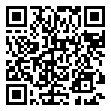• 分享
• 设计
免费设计
• 计算器
装修计算器
• 入驻
合作入驻
• 联系
联系我们
• 置顶
返回顶部